Europlanet Science Congress 2021
Virtual meeting
13 September – 24 September 2021
EPSC Abstracts
Vol. 15, EPSC2021-611, 2021
https://doi.org/10.5194/epsc2021-611
European Planetary Science Congress 2021

# A machine learning approach to classifying MESSENGER FIPS proton spectra

Matthew James et al.

Abstract

The entire dataset of 1-minute proton spectra provided by MESSENGER’s Fast Imaging Plasma Spectrometer (FIPS ) are each numerically fitted with a κ distribution function in order to provide estimates of proton density, n, and temperature, T , in the vicinity of Mercury’s magnetosphere. A collection of artificial neural networks (ANNs) are trained to assess the quality of the newly fitted distribution functions, with a final classification accuracy of 96%. Of the 223,282 spectra, ∼160,000 were classified as having “good” fitting κ distributions, ∼133,000 of which were measurements obtained from within the magnetosphere, and ∼18,000 were from the magnetosheath. The “good” magnetospheric densities and temperatures were subsequently used to create a 3D ANN-based model of the Hermean magnetosphere.

1. Introduction

Mass loading is an important factor in controlling the timescales over which processes such as wave propagation and reconnection can occur within a magnetized plasma. The speed at which magnetohydrodynamic (MHD) waves propagate is characterized by the local Alfvén velocity, vA , where vA=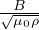, B is themagnetic field magnitude, ρ is the plasma mass density and μ0 is the permeability of free space. Fundamentally, the higher the local plasma mass density is–the slower waves propagate. Similarly, the beta of a plasma (β) depends on the density and temperature where β=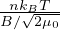and kB is the Boltzmann constant.

Magnetic reconnection between two adjacent magnetized plasmas is affected by the difference in plasma beta (∆β) between the two fluids, where a larger ∆β suppresses reconnection and thus a larger shear angle is required for reconnection to occur [2, 3]; conversely, low ∆β makes reconnection more favorable with small shear angles [3, 4].
The Fast Imaging Plasma Spectrometer (FIPS ) on board the MErcury Surface, Space ENvironment, GEochemistry and Ranging (MESSENGER ) spacecraft operated at Mercury for approximately 4 years (from March 2011 to April 2015) during the orbital phase of the mission. During this time, it sampled the ions from both within and outside of the Hermean magnetosphere, providing at least 1.25 million proton spectra every 10 or 60 s, depending upon the instrument mode. Many of those were burst-mode spectra and were combined in groups of 6 to form 1-minute average spectra, resulting in ∼220,000 60 s proton spectra.

In the work presented here, the entire dataset of 1-minute FIPS spectra are each numerically fitted with κ distribution functions, providing improved estimates of proton density and temperature over previous Maxwellian fits. The quality of the distribution function fits are assessed using artificial neural networks, resulting in a reduced dataset of more reliable plasma moments. Finally, new average and scalable 3D models of magnetospheric protons are presented using the new moments.

2. Fitting the Spectra

The κ-distribution function, fκ ,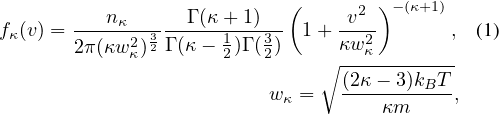where Γ(x) is the Gamma function, was fitted numerically to the FIPS spectra using the downhill-simplex method . This distribution function can be used to describe both plasmas in thermal equilibrium (i.e. Maxwellian, κ → ∞) and suprathermal plasmas (κ < 10).

Figure 1 shows an example of the κ distribution fit (pink) to a FIPS spectrum, compared to a Maxwellian fit (gray). The κ function fits well at all velocities in the FIPS spectrum, while the Maxwellian deviates significantly at higher energies.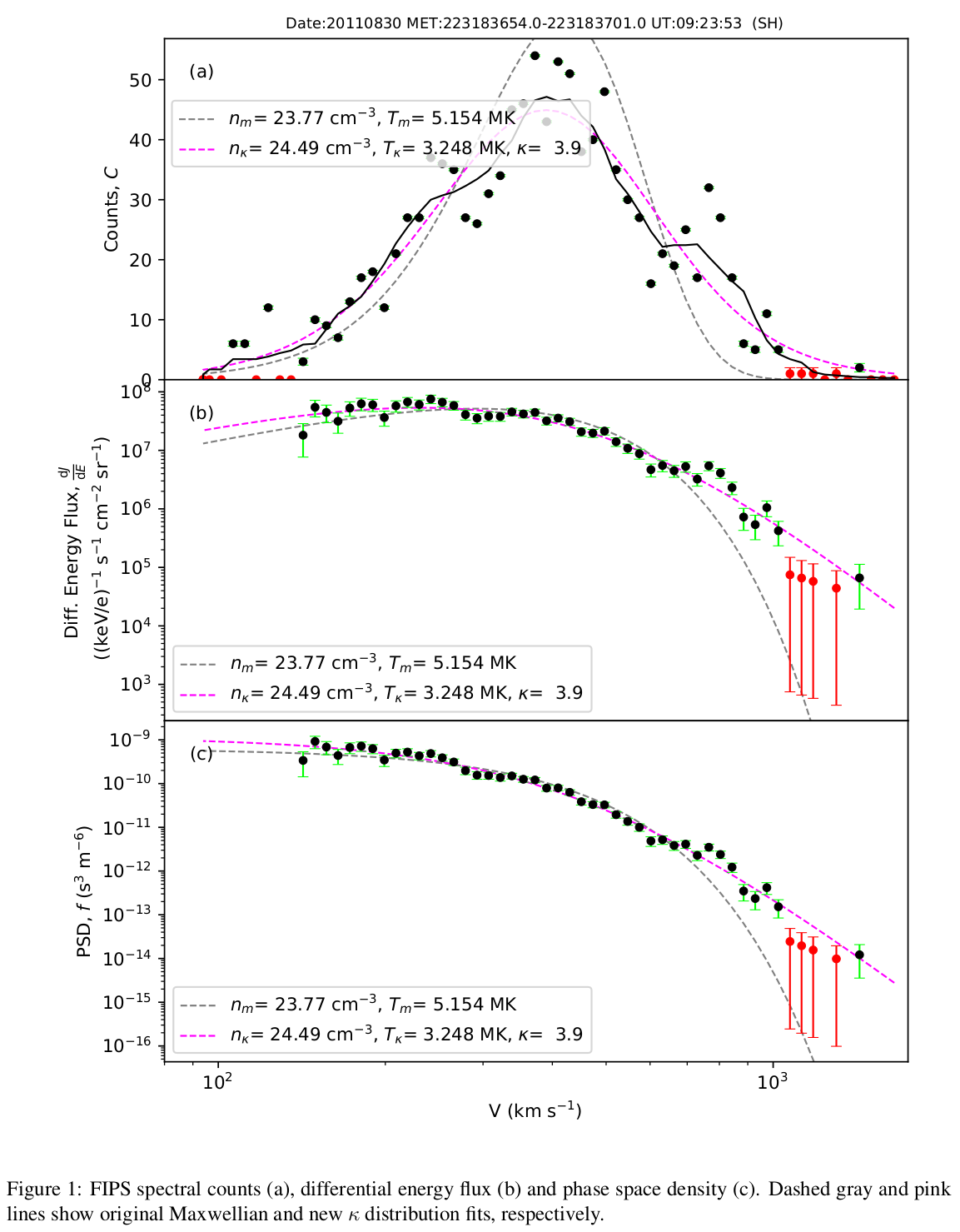3. Assessing Quality of Spectral Fit

Determining whether the κ distribution fit is a simple task to perform by eye, but would be a time consuming task to do for all 223,282 spectra, with potentially inconsistent results. One option would be to use a simple measure of goodness of fit (e.g. χ2 ), but this does not work very well with these data - so classification neural networks were used instead.
Initially, spectra were split into 8 equal parts, each of which was analyzed by one of 8 neural networks (see figure 2) – providing a probability that each section had a “good” distribution function fit. The 8 probabilities formed part of the input to the final ANN, along with other parameters such as ∆f (difference between the spectral fit and the data), which produced an overall probability that the spectral fit was reliable.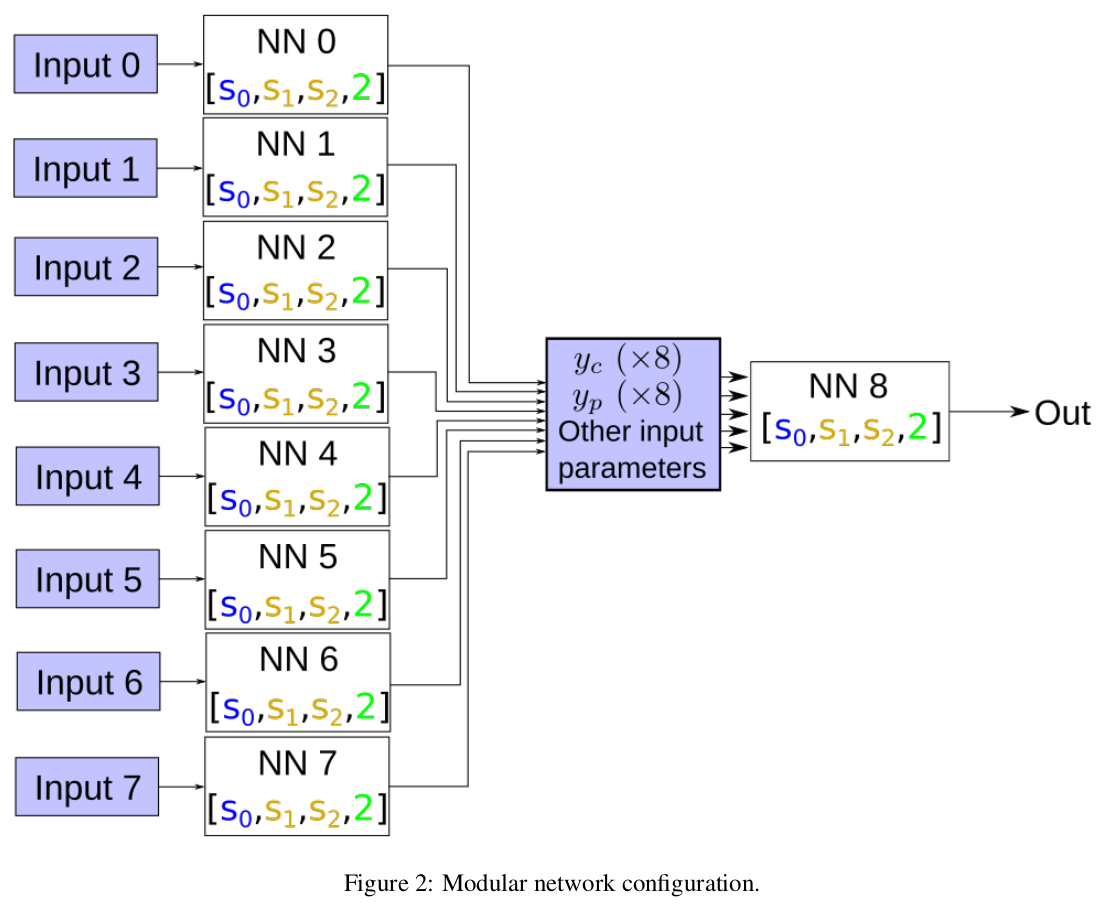4. 3D Proton Model

Figure 3 shows an overview of the new magnetospheric proton densities and temperatures in the equatorial plane and in the X-Z plane. The proton density appears to be lowest in the closed regions of the magnetosphere, and highest in the cusp, whereas the temperatures exhibit the inverse of this trend.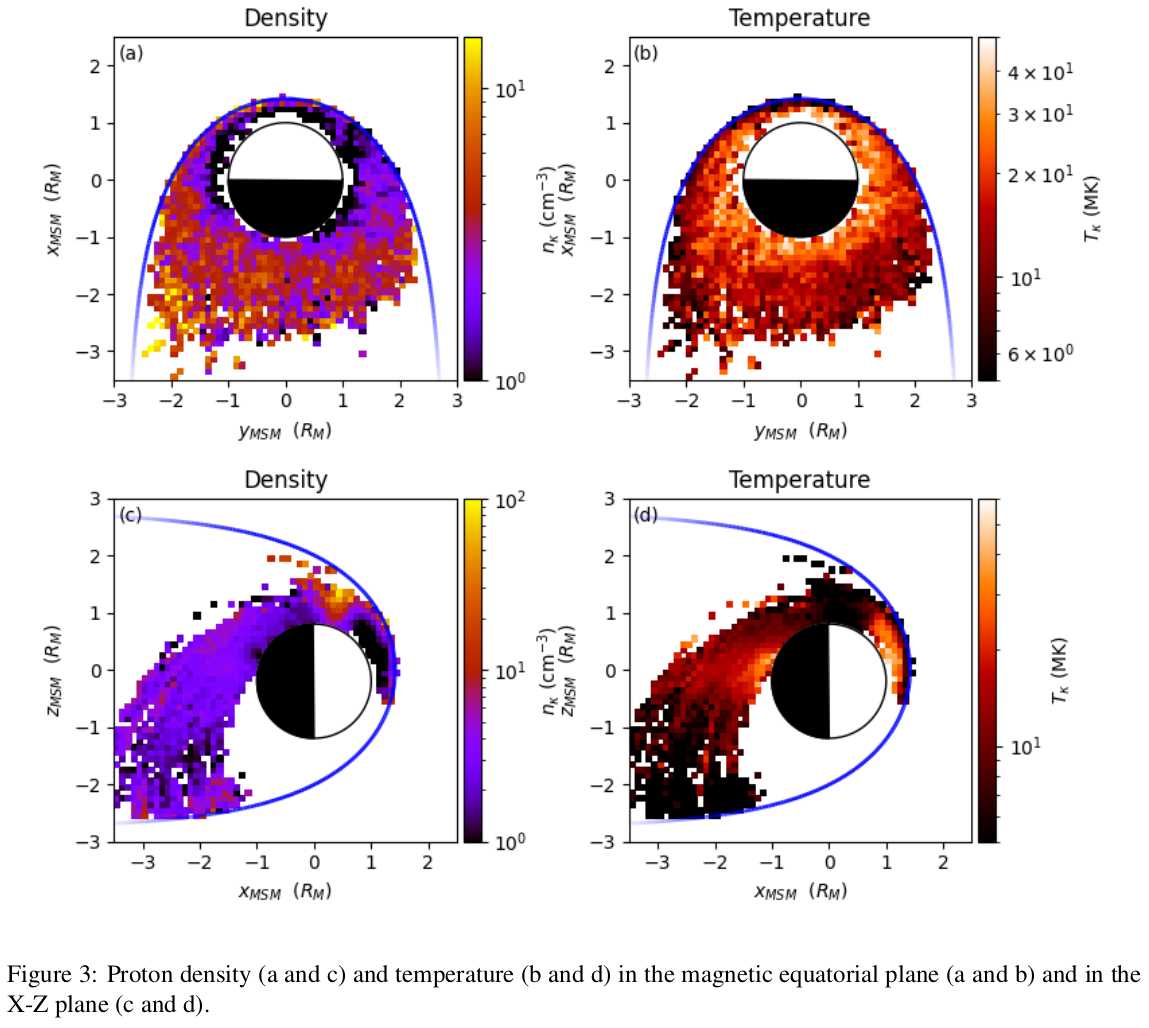Using the new density and temperature measurements as training data, and assuming that there is some hemispherical symmetry, a new 3D model was created using an ANN (see figure 4 a and b). The model was trained simply to map a Cartesian position vector as an input to density and temperature. The model may also be combined with the KT17 magnetic field model  to provide estimates of the upper limit to the Alfvén speed (figure 4c) and the proton contribution to plasma β (figure 4d). A scalable model was also produced, where the magnetopause standoff distance was used as an extra ANN input.